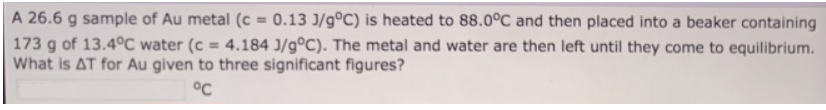Chemistry Calorimetry Solution: A 26.6 g sample of Au metal (c = 0.13 J/g°C) is he...

# Solution: A 26.6 g sample of Au metal (c = 0.13 J/g°C) is heated to 88.0°C and then placed into a beaker containing 173 g of 13.4 °C water (c = 4.184 J/g°C). The metal and water are then left until they come to equilibrium. What is ΔT for Au given to three significant figures?

Problem

A 26.6 g sample of Au metal (c = 0.13 J/g°C) is heated to 88.0°C and then placed into a beaker containing 173 g of 13.4 °C water (c = 4.184 J/g°C). The metal and water are then left until they come to equilibrium. What is ΔT for Au given to three significant figures?View Complete Written Solution